General Formula: Matrix Inversion Lemma

Let,, and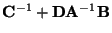be non-singular square matrices; then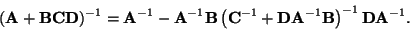General Formula: Matrix Inversion in Block form

Let amatrix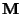be partitioned into a block form: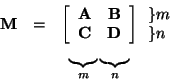where the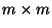matrixandmatrix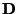are invertible. Then we have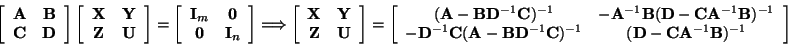It can be proved that the above two matrix expressions forare equivalent.

Special Case 1

Let a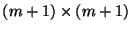matrixbe partitioned into a block form: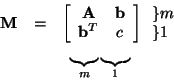Then the inverse ofis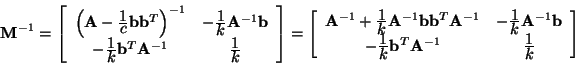where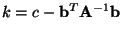.

Special Case 2

Suppose that we have a given matrix equation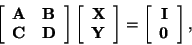(1)

whereandare invertible matrices and all matrices are of compatible dimensions in the above equation. Please find the matricesand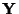in terms of the given matrices,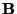,, and.